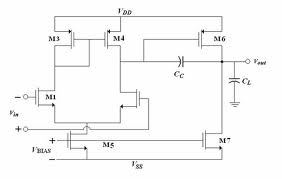# output levels in the two stage op-amp

Status
Not open for further replies.

#### Junus2012

Dear all

for the attached traditional op-amp, I am simulating the output maximum levels from the DC simulation by sweeping one one of the inputs. I have found that the minimum output is zero and the maximum is VDD.

Here is my question, for the output it is ok to approach VDD when the current in M6 is zero. but for M7 the current is never being zero as it mirror the current from the current source so how the minimum output is getting zero ????

Thank you

- - - Updated - - -

I forgot to attach the image, here it isfor the output it is ok to approach VDD when the current in M6 is zero.

The opposite is the case - when current in M6 is zero, the output will be zero volts (pulled down by M7). The output reaches VDD because M6 can source a lot more current than M7 sinks, I would assume.

Keith

Hello Keith
Thank you to reply my post

I would say No, M6 is pull the output toward VDD, so if I6=0 then the voltage drop over the transistor is zero so the output is
VDD-0 = VDD

M7 pull down the output to zero if the voltage drop over it zero, but since the voltage drop cant be zero over this transistor as it is current source, therefore I am wondering how the output is going to zero from the DC characteristics
The opposite is the case - when current in M6 is zero, the output will be zero volts (pulled down by M7). The output reaches VDD because M6 can source a lot more current than M7 sinks, I would assume.

Keith

I agree M6 is to pull the output to VDD but it will only do that when there is current flowing through it, not when the current in M6 is zero.

As you have a simulation of the circuit you can probe the currents in M6 and M7 and see that what I say is correct.

Keith

Status
Not open for further replies.